Trigonometry : Solving Word Problems with Trigonometry

Example Questions

← Previous 1

Example Question #1 : Solving Word Problems With Trigonometry

While waiting for your sister to finish her bungee jump, you decide to figure out how tall the platform she is jumping off is. You are standingfeet from the base of the platform, and the angle of elevation from your position to the top of the platform is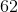degrees. How many feet tall is the platform?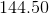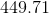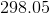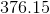Explanation:

You can draw the following right triangle using the information given by the question: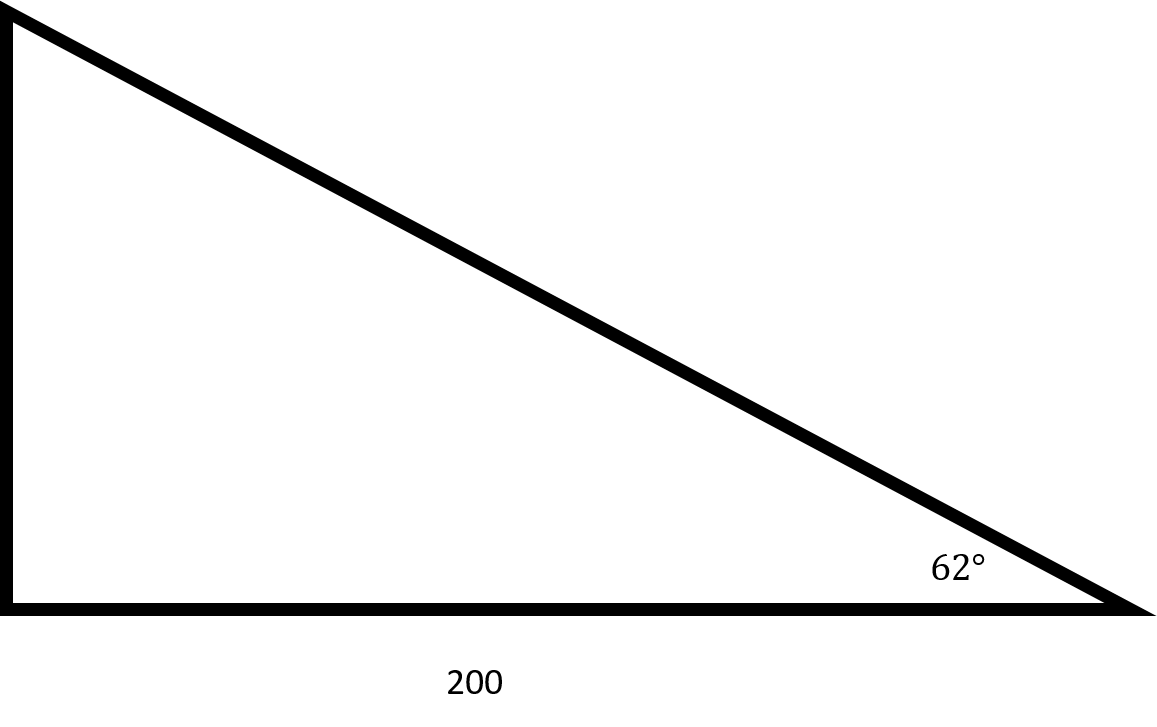Since you want to find the height of the platform, you will need to use tangent.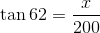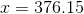Make sure to round toplaces after the decimal.

Example Question #2 : Solving Word Problems With Trigonometry

When the angle of elevation of the sun isdegrees, a flagpole casts a shadow that isfeet long. In feet, how tall is the flagpole?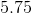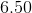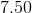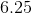Explanation:

You can draw the following right triangle from the information given by the question.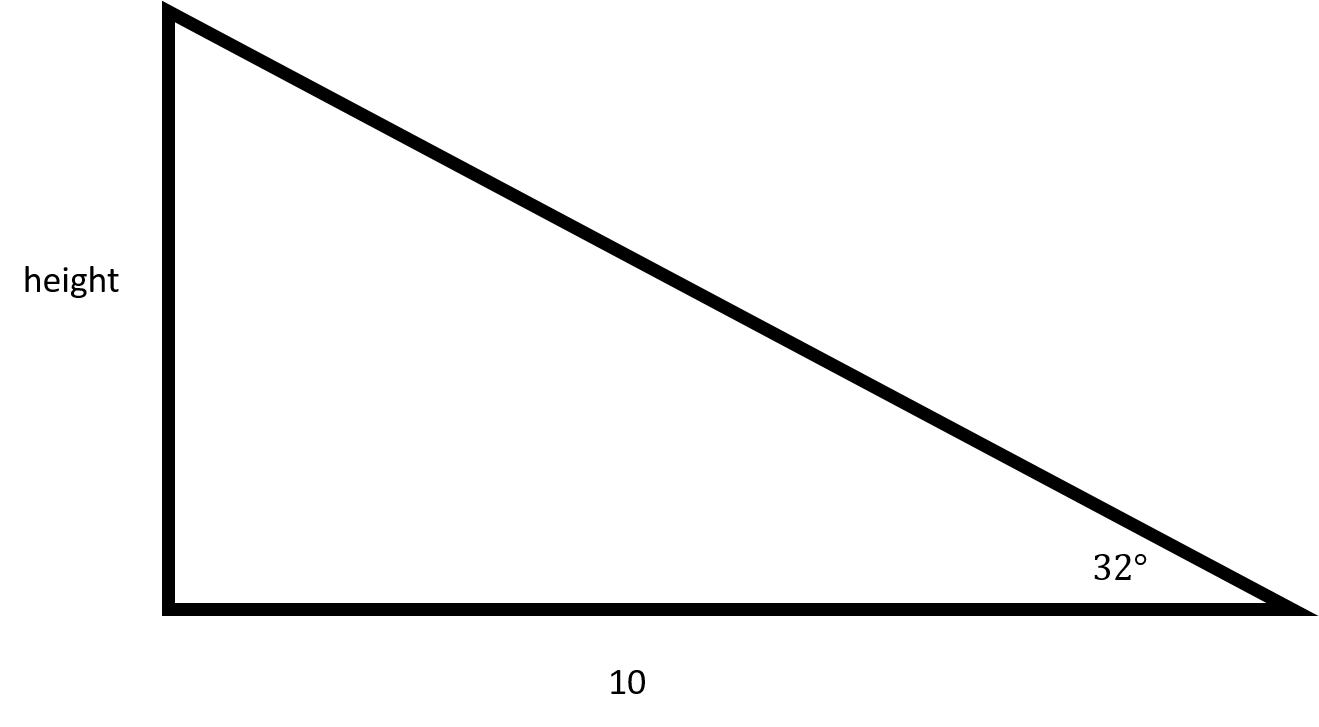In order to find the height of the flagpole, you will need to use tangent.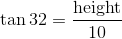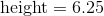Make sure to round toplaces after the decimal.

The flagpole isfeet tall.

Example Question #3 : Solving Word Problems With Trigonometry

A ladder that isfeet long is resting against the side of a house at an angle ofdegrees. In feet, how far up the side of the house does the ladder reach?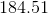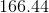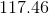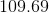Explanation:

You can draw the following right triangle from the information given in the question: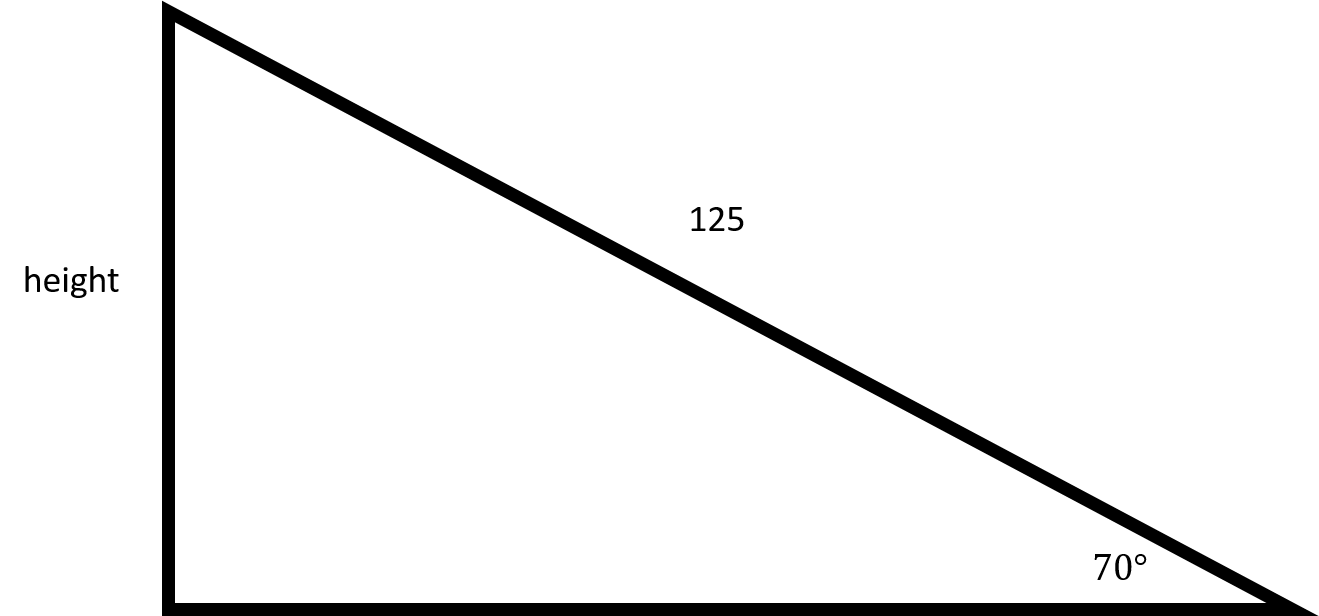In order to find out how far up the ladder goes, you will need to use sine.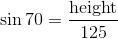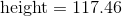Example Question #1 : Solving Word Problems With Trigonometry

In right triangle ABC, where angle A measures 90 degrees, side AB measures 15 and side AC measures 36, what is the length of side BC?This triangle cannot exist.Explanation:

This triangle can exist. Since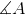is a right angle, we can use the Pythagorean Theorem, whereis the hypoteneuse: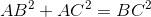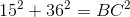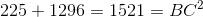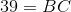Example Question #5 : Solving Word Problems With Trigonometry

A support wire is anchored 10 meters up from the base of a flagpole, and the wire makes a 25o angle with the ground. How long is the wire, w? Round your answer to two decimal places.

28.31 meters

25 meters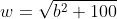23.81 meters

21.83 meters

23.81 meters

Explanation:

To make sense of the problem, start by drawing a diagram. Label the angle of elevation as 25o, the height between the ground and where the wire hits the flagpole as 10 meters, and our unknown, the length of the wire, as w.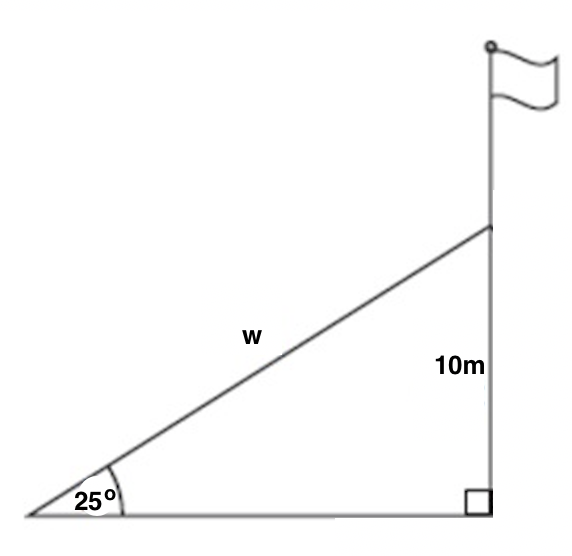Now, we just need to solve for w using the information given in the diagram. We need to ask ourselves which parts of a triangle 10 and w are relative to our known angle of 25o. 10 is opposite this angle, and w is the hypotenuse. Now, ask yourself which trig function(s) relate opposite and hypotenuse. There are two correct options: sine and cosecant. Using sine is probably the most common, but both options are detailed below.

We know that sine of a given angle is equal to the opposite divided by the hypotenuse, and cosecant of an angle is equal to the hypotenuse divided by the opposite (just the reciprocal of the sine function). Therefore: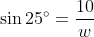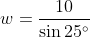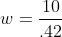(Use a calculator in degree mode to find that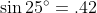after rounding to two decimal places)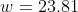meters

To solve this problem instead using the cosecant function, we would get: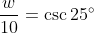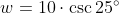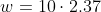(Use a calculator in degree mode to find that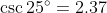after rounding to two decimal places)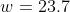meters

The reason that we got 23.7 here and 23.81 above is due to differences in rounding in the middle of the problem.

Please note that the answer choiceis correct based on the Pythagorean Theorem, but does not use all of the provided info to find an exact solution rounded to two decimal places.

Example Question #6 : Solving Word Problems With Trigonometry

When the sun is 22o above the horizon, how long is the shadow cast by a building that is 60 meters high?

162 meters

65 meters

150 meters

24 meters

82 meters

150 meters

Explanation:

To solve this problem, first set up a diagram that shows all of the info given in the problem.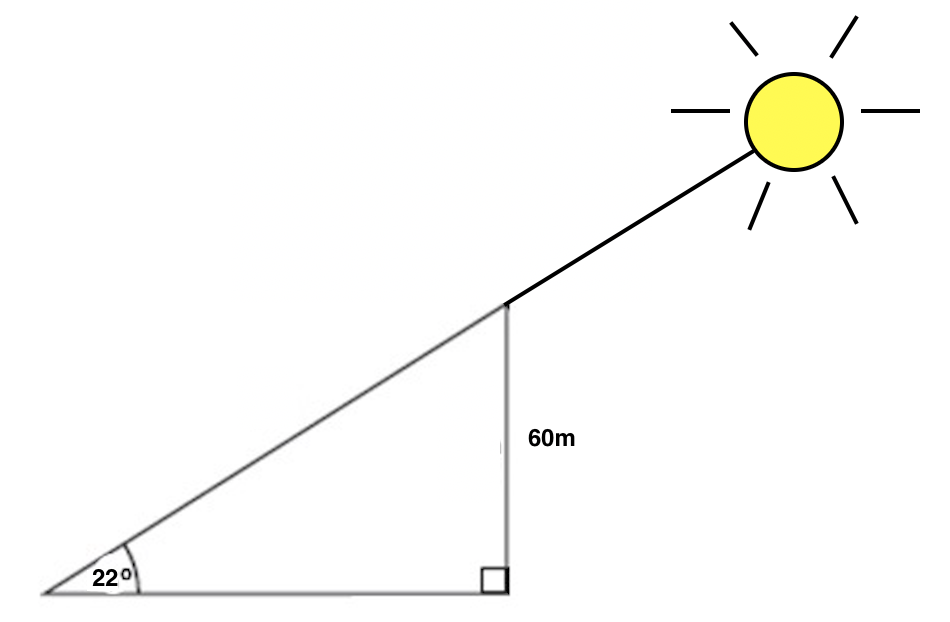Next, we need to interpret which side length corresponds to the shadow of the building, which is what the problem is asking us to find. Is it the hypotenuse, or the base of the triangle? Think about when you look at a shadow. When you see a shadow, you are seeing it on something else, like the ground, the sidewalk, or another object. We see the shadow on the ground, which corresponds to the base of our triangle, so that is what we'll be solving for. We'll call this base b.

Next, think about which trig functions relate our known angle, 22o, to the base (or adjacent) and the opposite sides of the triangle. If you thought tangent (or cotangent), you are correct! We know that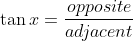and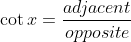. For simplicity's sake, we'll use tangent to solve this problem. We have: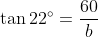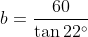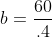(Use a calculator and round to two places to find that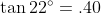)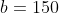meters

Therefore the shadow cast by the building is 150 meters long.

If you got one of the incorrect answers, you may have used sine or cosine instead of tangent, or you may have used the tangent function but inverted the fraction (adjacent over opposite instead of opposite over adjacent.)

Example Question #31 : Right Triangles

From the top of a lighthouse that sits 105 meters above the sea, the angle of depression of a boat is 19o. How far from the boat is the top of the lighthouse?

318.18 meters

110.53 meters

36.15 meters

423.18 meters

318.18 meters

Explanation:

To solve this problem, we need to create a diagram, but in order to create that diagram, we need to understand the vocabulary that is being used in this question. The following diagram clarifies the difference between an angle of depression (an angle that looks downward; relevant to our problem) and the angle of elevation (an angle that looks upward; relevant to other problems, but not this specific one.) Imagine that the top of the blue altitude line is the top of the lighthouse, the green line labelled GroundHorizon is sea level, and point B is where the boat is.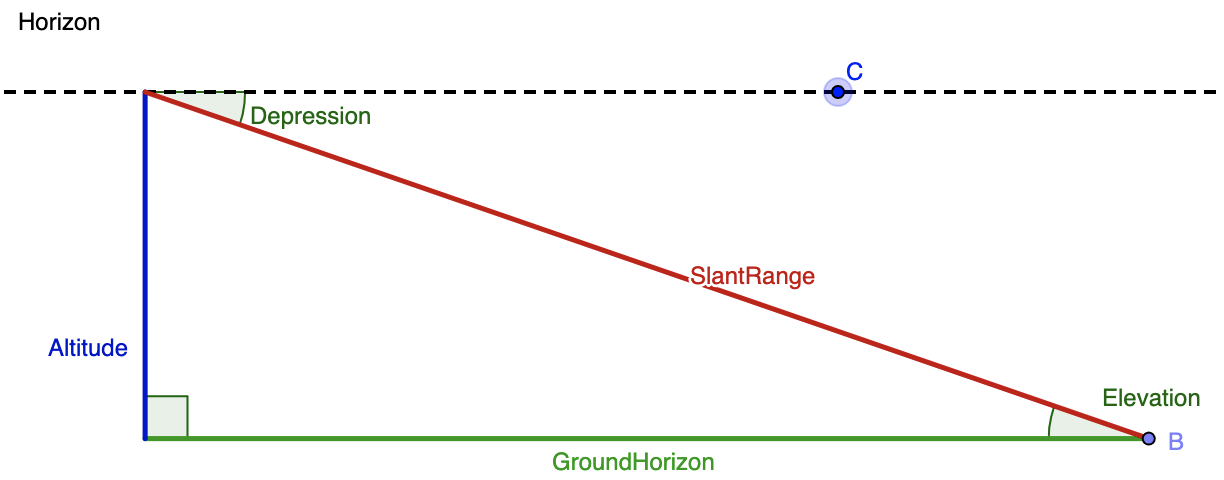Merging together the given info and this diagram, we know that the angle of depression is 19o and and the altitude (blue line) is 105 meters. While the blue line is drawn on the left hand side in the diagram, we can assume is it is the same as the right hand side. Next, we need to think of the trig function that relates the given angle, the given side, and the side we want to solve for. The altitude or blue line is opposite the known angle, and we want to find the distance between the boat (point B) and the top of the lighthouse. That means that we want to determine the length of the hypotenuse, or red line labelled SlantRange. The sine function relates opposite and hypotenuse, so we'll use that here. We get: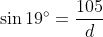(where d is the distance between the top of the lighthouse and the boat)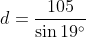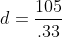(using a calculator in degree mode and rounding to two digits, we get that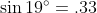)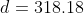meters

Example Question #32 : Right Triangles

Angelina just got a new car, and she wants to ride it to the top of a mountain and visit a lookout point. If she drives 4000 meters along a road that is inclined 22o to the horizontal, how high above her starting point is she when she arrives at the lookout?

10677.87 meters

3708.74 meters

9.37 meters

1616.1 meters

1480 meters

1480 meters

Explanation:

As with other trig problems, begin with a sketch of a diagram of the given and sought after information.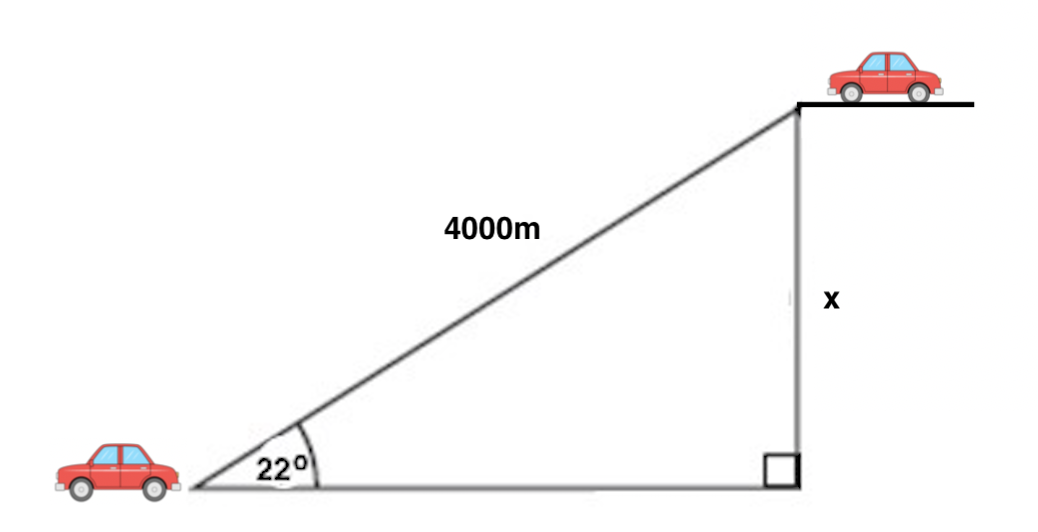Angelina and her car start at the bottom left of the diagram. The road she is driving on is the hypotenuse of our triangle, and the angle of the road relative to flat ground is 22o. Because we want to find the change in height (also called elevation), we want to determine the difference between her ending and starting heights, which is labelled x in the diagram. Next, consider which trig function relates together an angle and the sides opposite and hypotenuse relative to it; the correct one is sine. Then, set up: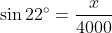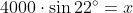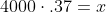(using a calculator in degree mode and rounding to two decimals we get that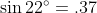)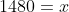Therefore the change in height between Angelina's starting and ending points is 1480 meters.

Example Question #33 : Right Triangles

Two buildings with flat roofs are 50 feet apart. The shorter building is 40 feet tall. From the roof of the shorter building, the angle of elevation to the edge of the taller building is 48o. How high is the taller building?

73.5 feet

55.5 feet

40 feet

77.2 feet

95.5 feet

95.5 feet

Explanation:

To solve this problem, let's start by drawing a diagram of the two buildings, the distance in between them, and the angle between the tops of the two buildings. Then, label in the given lengths and angle.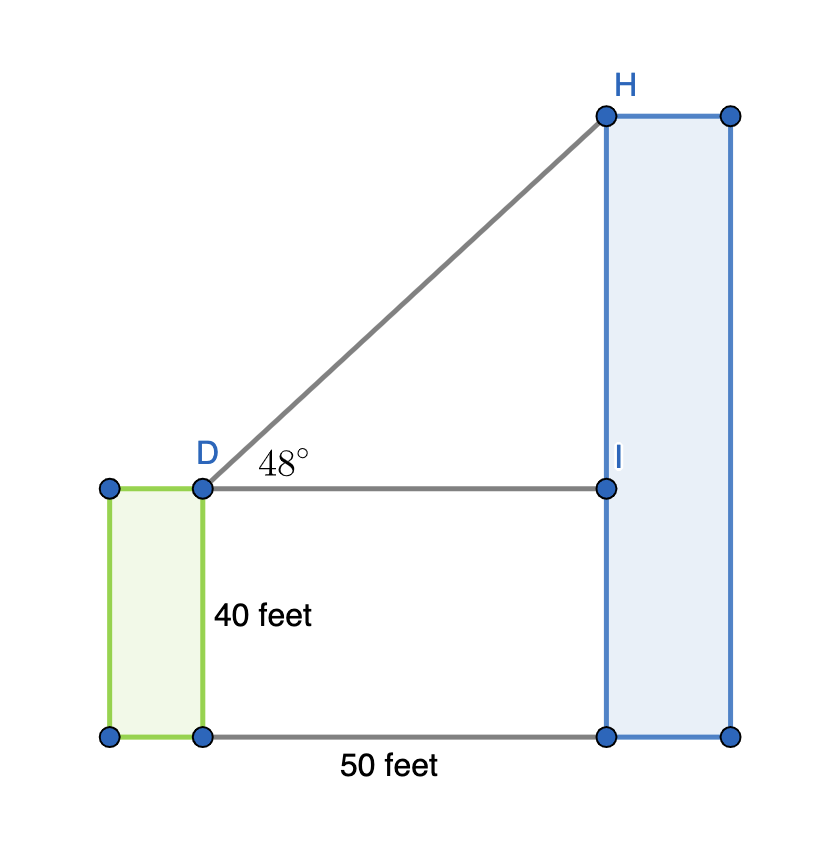We are being asked to find the height of the taller building, but this diagram does not provide a triangle that has as one of its sides the entire height of the larger (rightmost and blue) building. However, we can instead find the distance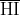, and then add that to the 40 foot height of the shorter building to find the entire height of the taller building. Start by finding: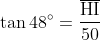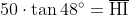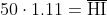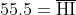Remember that this is not the full height of the larger building. To find that, we need to add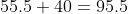feet. Therefore, the taller building is 95.5 feet tall.

Example Question #34 : Right Triangles

Two buildings with flat roofs are 80 feet apart. The shorter building is 55 feet tall. From the roof of the shorter building, the angle of elevation to the edge of the taller building is 32o. How high is the taller building?

97.4 feet

129.6 feet

122.8 feet

49.6 feet

104.6 feet

104.6 feet

Explanation:

To solve this problem, let's start by drawing a diagram of the two buildings, the distance in between them, and the angle between the tops of the two buildings. Then, label in the given lengths and angle.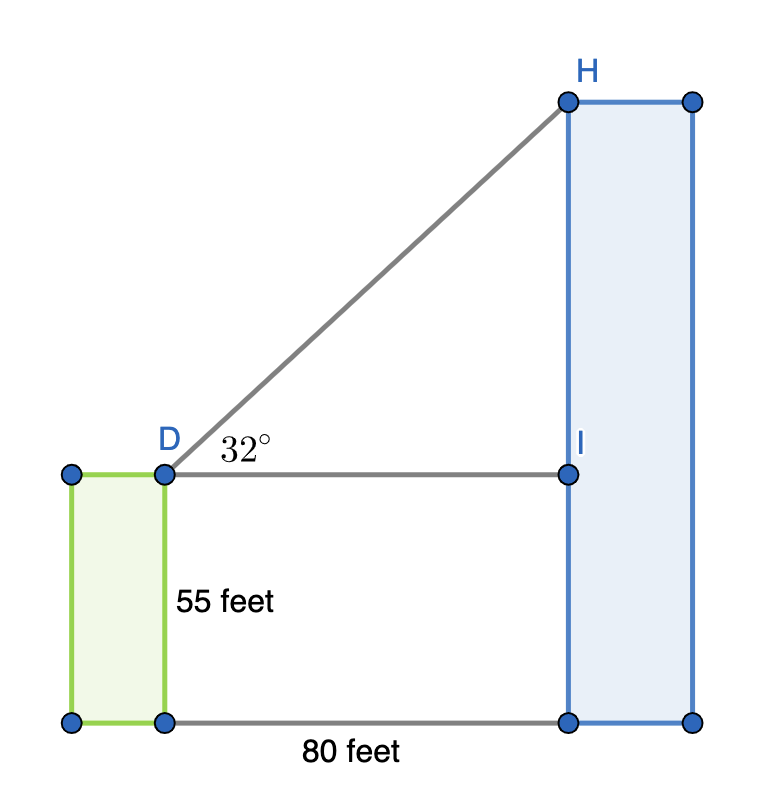We are being asked to find the height of the taller building, but this diagram does not provide a triangle that has as one of its sides the entire height of the larger (rightmost and blue) building. However, we can instead find the distance, and then add that to the 40 foot height of the shorter building to find the entire height of the taller building. Start by finding: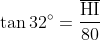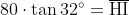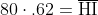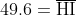Remember that this is not the full height of the larger building. To find that, we need to add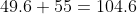feet. Therefore, the taller building is 104.6 feet tall.

← Previous 1